### Home > CALC > Chapter 1 > Lesson 1.4.1 > Problem1-142

1-142.
1. For each function, use algebra to identify all holes and asymptotes. Homework Help ✎

1.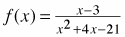2.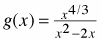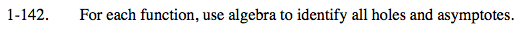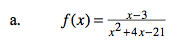Factor the denominator. This will help you determine the location of vertical asymptotes and holes.

If there is a 0 in the denominator, it indicates either a hole or a vertical asymptote. Holes exist where the 0 'cancels out.' (Don't forget to find the y-value as well.) Vertical asymptotes exist where it doesn't.

If there is a horizontal asymptote, then it is also the end behavior. If there isn't, you will have to long divide to find the end behavior. Horizontal asymptotes can be found after 'cancelling' and simplifying the fraction... if y→(a constant) as x→∞, then that constant is the horizontal asymptote.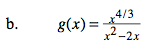See part (a) for help.